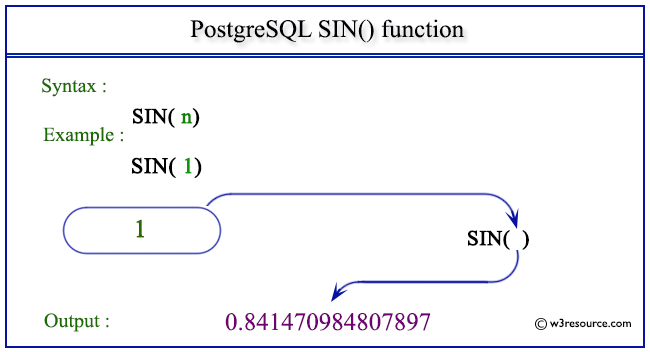# PostgreSQL SIN() function

## SIN() function

The PostgreSQL sin() function is used to return the sine of a given argument.

Syntax:

```sin(a)
```

PostgreSQL Version: 9.3

Pictorial presentation of PostgreSQL SIN() functionExample 1: PostgreSQL SIN() function

Code:

``````SELECT SIN(0) AS "Sin (0)";
```
```

Sample Output:

``` Sin (0)
---------
0
(1 row)
```

Example 2: PostgreSQL SIN() function

Code:

``````SELECT SIN(1) AS "Sin (1)";
```
```

Sample Output:

```      Sin (1)
-------------------
0.841470984807897
(1 row)
```

Example 2: PostgreSQL SIN() function

Code:

``````SELECT SIN(-1) AS "Sin (-1)";
```
```

Sample Output:

```      Sin (-1)
--------------------
-0.841470984807897
(1 row)
```

Previous: SIGN function
Next: SQRT function

﻿